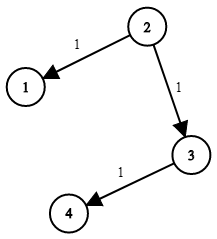743. Network Delay Time

You are given a network of `n` nodes, labeled from `1` to `n`. You are also given `times`, a list of travel times as directed edges `times[i] = (ui, vi, wi)`, where `ui` is the source node, `vi` is the target node, and `wi` is the time it takes for a signal to travel from source to target.

We will send a signal from a given node `k`. Return the time it takes for all the `n` nodes to receive the signal. If it is impossible for all the `n` nodes to receive the signal, return `-1`.

Example 1:```Input: times = [[2,1,1],[2,3,1],[3,4,1]], n = 4, k = 2
Output: 2
```

Example 2:

```Input: times = [[1,2,1]], n = 2, k = 1
Output: 1
```

Example 3:

```Input: times = [[1,2,1]], n = 2, k = 2
Output: -1
```

Constraints:

• `1 <= k <= n <= 100`
• `1 <= times.length <= 6000`
• `times[i].length == 3`
• `1 <= ui, vi <= n`
• `ui != vi`
• `0 <= wi <= 100`
• All the pairs `(ui, vi)` are unique. (i.e., no multiple edges.)

743. Network Delay Time
``````struct Solution;
use std::collections::VecDeque;

impl Solution {
fn network_delay_time(times: Vec<Vec<i32>>, n: i32, k: i32) -> i32 {
let n = n as usize;
let k = k as usize - 1;
let mut graph: Vec<Vec<(usize, i32)>> = vec![vec![]; n];
for time in times {
let u = time as usize - 1;
let v = time as usize - 1;
let t = time;
graph[u].push((v, t));
}
let mut visited = vec![std::i32::MAX; n];
let mut queue = VecDeque::new();
visited[k] = 0;
queue.push_back(k);
while let Some(u) = queue.pop_front() {
for &(v, t) in &graph[u] {
if t + visited[u] < visited[v] {
visited[v] = t + visited[u];
queue.push_back(v);
}
}
}
let max = visited.into_iter().max().unwrap();
if max == std::i32::MAX {
-1
} else {
max
}
}
}

#[test]
fn test() {
let times = vec_vec_i32![[2, 1, 1], [2, 3, 1], [3, 4, 1]];
let n = 4;
let k = 2;
let res = 2;
assert_eq!(Solution::network_delay_time(times, n, k), res);
}
``````